# KVPY-SX 2017 Physics Paper with Solutions

KVPY SX is an examination conducted for students in Class 12 to encourage them to pursue a career in research. The exam aims at providing scholarships to the students who score well in the examination. The students can crack the examination by practising previous years’ question papers. On this page, we have answered the questions asked in the KVPY SX 2017 Physics paper. The question paper includes questions from various physics topics like current electricity, heat and thermodynamics, optics, atomic physics, etc.

### KVPY SX 2017 - Physics

Question 1: The magnitude of the acceleration of the electron in the nth orbit of the hydrogen atom is aH and that of singly ionized helium atom is aHe. The ratio aH:aHe is

1. a. 1 : 8
2. b. 1 : 4
3. c. 1 : 2
4. d. dependent on n

Solution:

The centripetal acceleration of electron in nth orbit,

$a_{n}=\frac{v_{n}^{2}}{r_{n}}$

an = acceleration of electron in nth orbit

n = integer, z = atomic number

Since, $v_{n}\propto \frac{z}{n}$ and $r_{n}\propto \frac{n^{2}}{z}$

$\Rightarrow a_{n}\propto \frac{z^{3}}{n^{4}}$

So, we get

$\frac{a_{H}}{a_{_{He}}}=\frac{Z^{3}_{H}}{Z^{3}_{He}}$

zH = atomic number of hydrogen

zHe = atomic number of helium

Given: zHe = 2 and zH = 1

$\Rightarrow \frac{a_{H}}{a_{_{He}}}=\frac{1^{3}}{2^{3}}=\frac{1}{8}$

Question 2: A carrot looks orange in colour because of the β carotene molecule in it. This means that the β carotene molecule absorbs light of wavelengths

1. a. longer than 550 nm
2. b. shorter than 550 nm
3. c. longer than 700 nm
4. d. shorter than 700 nm

Solution:

By the help of VIBGYOR

VIBG:400nm:Absorbed

YOR: 700 nm:Reflected

As we know in VIBGYOR, the wavelength of a different colour is different in a specific region. So, O stood for orange and observed the wavelength shorts than 550 nm. The concept behind it is that the colour that we see in the reflected specific wavelength and rest all the complementary colours are absorbed. As we can see that in the question it is given that we are perceiving the orange colour then this means that only the orange wavelength is the reflected one. And the wavelength of yellow, orange, and red lies above 700 nm. So the complementary absorbed wavelengths are violet, indigo, blue, green and all these wavelengths lie below 550 nm.

Question 3: If some charge is given to a solid metallic sphere, the field inside remains zero and by Gauss's law all the charge resides on the surface. Suppose now that Coulomb’s force between two charges varies as 1/r3. Then, for a charged solid metallic sphere

1. a. Field inside will be zero and charge density inside will be zero.
2. b. Field inside will not be zero and charge density inside will not be zero.
3. c. Field inside will not be zero and charge density inside will be zero.
4. d. Field inside will be zero and charge density inside will not be zero.

Solution:

According to questions,

Coulomb’s force is

$F\propto \frac{1}{r^{3}}$

Then Gauss law will not follow.

$\phi \neq \frac{q_{in}}{\varepsilon _{0}}$

If condition is static thenWhere, $\phi \rightarrow$ field lines per unit area.

If electric field is zero then field lines will also be zero.

$\phi =0$

$\Rightarrow \phi \neq \frac{q_{in}}{\varepsilon _{0}}$

Therefore, $\frac{q_{in}}{\varepsilon _{0}}\neq 0$

So, charge inside will not be zero and charge density also will not be zero. But electric field is zero inside.

Question 4: Using dimensional analysis the resistivity in terms of fundamental constants h, me, c, e, can be expressed as

1. a. $\frac{h}{\varepsilon _{0}m_{e}ce^{2}}$
2. b. $\frac{\varepsilon _{0}m_{e}ce^{2}}{h}$
3. c. $\frac{h^{2}}{m_{e}ce^{2}}$
4. d. $\frac{m_{e}\varepsilon _{0}}{ce^{2}}$

Solution:

Since, Electric field = Resistivity × Current density

E = ρ × J

$\Rightarrow \rho =\frac{E}{J}$ …(1)

Dimension of E and J are

Since, [E] = [MLT–3I–1] and J = [IL–2]

Put these dimension in eq.(1)

$\left [ \rho \right ]=\frac{\left [ MLT^{-3}I^{-1} \right ]}{\left [ IL^{-2} \right ]}=\left [ ML^{3}T^{-3}I^{-2} \right ]$

Since, h = [ML2T–1]

me = [M]

C = [LT–1]

εo = [M–1L–3T4I2]

e = [IT]

From question

ρ= haMebCcε0dee

Now, put all the dimensions in equation

[ML3T–3I–2] = [ML2T–1]a[M]b[LT–1]c[M–1L–3T4I2]d[IT]e

Now compare

[M1L3T–3I–2] = [Ma+b–d][L 2a+c–3d][T –a–c+4d+e][I 2d+e]

Now compare powers of LHS and RHS,

1 = a + b – d …(1)

3 = 2a + c – 3d …(2)

–3 = –a – c + 4d + e …(3)

–2 = 2d + e …(4)

By solving we get, d = 0, a = 2, b = –1, c = –1, e = –2

$\rho =\frac{h^{2}}{m_{e}Ce^{2}}$

Question 5: Consider a bowl filled with water on which some black pepper powder has been sprinkled uniformly. Now a drop of liquid soap is added at the centre of the surface of water. The picture of the surface immediately after this will look like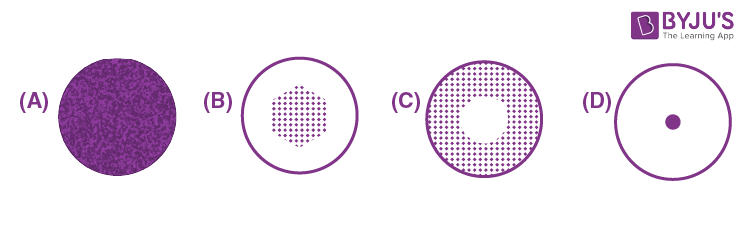Solution:

The water surface exerts a surface tension when the pepper powder is sprinkled uniformly on the water surface, the powder particles remain at their position. But as soon as a drop of liquid soap is added at the center of water surface, surface tension of water breaks at the center, so the remaining part of water exerts a net surface tension outwards and the water spreads outwards taking the powder particles outwards along with it as shown in the figure C.

Question 6: It was found that the refractive index of material of a certain prism varied as 1.5 + 0.004/ λ2, where λ is the wavelength of light used to measure the refractive index. The same material was then used to construct a thin prism of apex angle 10°. Angles of minimum deviation (δm) of the prism were recorded for the sources with wavelengths λ1 and λ2 respectively. Then

1. a. $\delta _{m}(\lambda _{1})< \delta _{m}(\lambda _{2}) if \lambda _{1}< \lambda _{2}$
2. b. $\delta _{m}(\lambda _{1})> \delta _{m}(\lambda _{2}) if \lambda _{1}> \lambda _{2}$
3. c. $\delta _{m}(\lambda _{1})> \delta _{m}(\lambda _{2}) if \lambda _{1}< \lambda _{2}$
4. d. $\delta _{m}$ is the same in both the cases

Solution:

Angle of minimum deviation,

δm = (µ – 1)A

δm ∝ µ

µ = refractive index

So, more is the refractive index of the material,

Lesser is the deviation.

$\delta _{m}\propto \frac{1}{\lambda ^{2}}$ (λ = wavelength)

Also, more is the wavelength of light, lesser is the refractive index of material.

δm1)> δm2) if λ 1< λ 2

λ 2> λ 1 if (δm)1> (δm)2

Question 7: Two circularly shaped linear polarisers are placed coaxially. The transmission axis of the first polarizer is at 30° from the vertical while the second one is at 60°, both in the clockwise sense. If an unpolarised beam of light of intensity I = 20 W/m2 is incident on this pair of polarisers, then the intensities I1 and I2 transmitted by the first and the second polarisers, respectively, will be close to

1. a. I1 = 10.0 W/m2 and I2 = 7.5 W/m2
2. b. I1 = 20.0 W/m2 and I2 = 15 W/m2
3. c. I1 = 10.0 W/m2 and I2 = 8.6 W/m2
4. d. I1 = 15.0 W/m2 and I2 = 0.0 W/m2

Solution:

Intensity of unpolarized light, I = 20 w/m2

Intensity of light passing through first polarizer,

I1 = I/2 = 20/2 = 10 w/m2

Angle between transmission axis of two polarizer, θ= 60° – 30° = 30°

Intensity of light passing through second polarizer,

I2 = I1cos2 θ

I2 = 10 × (3/4)

I2 = 7.5 w/m2

Question 8: An electron in an electron microscope with initial velocity $v_{0}\hat{i}$ enters a region of a stray transverse electric field $E_{0}\hat{j}$ . The time taken for the change in its de-Broglie wavelength from the initial value of λ to λ /3 is proportional to

1. a. E0
2. b. 1/E0
3. c. $\frac{1}{\sqrt{E_{0}}}$
4. d. $\sqrt{E_{0}}$

Solution: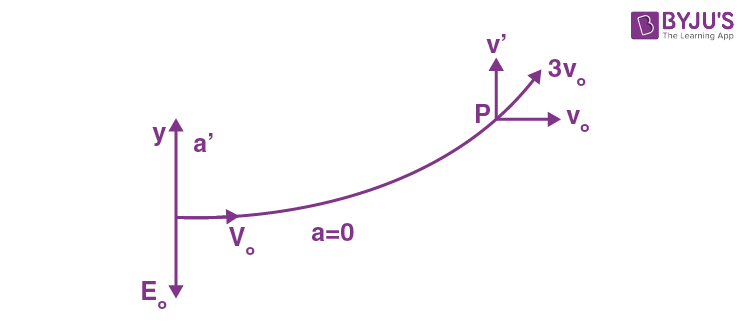Path traced by the electron is shown in the figure such that electron reaches the point P when its de-Broglie wavelength becomes λ/3 .

Acceleration of electron is zero in x-direction.

Acceleration of electron in y-direction a’ = eE0/m

Velocity of electron initially, vo = h/ λ

At P, vp = h/( λ/3) = 3vo

So, velocity is in y direction,

$v'=\sqrt{(v')^{2}-v_{0}^{2}}=\sqrt{9v_{0}^{2}-v_{0}^{2}}$

$v'=2\sqrt{2}$

Using v’=a’t

Therefore, $2\sqrt{2}v_{0}=\frac{eE_{0}}{m}t$ (Therefore, a = F/m , F= qE =eE so a= eE/m)

We get, $t=\frac{2\sqrt{2}v_{0}m}{eE_{0}}$

$\Rightarrow t\propto \frac{1}{E_{0}}$

Question 9: A bird sitting on a single high tension wire does not get electrocuted because

1. a. The circuit is not complete.
2. b. The bird feet has an insulating covering.
3. c. Capacitance of the bird is too small and the line frequency is too small.
4. d. Resistance of the bird is too high

Solution:

The answer to this question has to be explained with the context of how electricity flows. It will always move along the path of least resistance and flows best through conductors. The copper in electrical wires is a great conductor, birds are not good conductors. That’s one reason they don’t get shocked when they sit on electrical wires. The energy bypasses the birds and keeps flowing along the wire instead. There’s another reason why birds can sit on a wire without getting shocked. There’s no voltage difference in a single wire. For electrons to move, there must be what scientists call a difference in electrical potential. For example, energy flows from an area of high voltage to areas of low voltage. If it flows through a single power line at 35,000 volts, it will continue along the path of least resistance. That means it’ll bypass birds because there’s no difference in electrical potential.

Question 10: A positive charge q is placed at the centre of a neutral hollow cylindrical conducting shell with its cross-section as shown in the figure belowWhich one of the following figures correctly indicates the induced charge distribution on the conductor (ignore edge effects).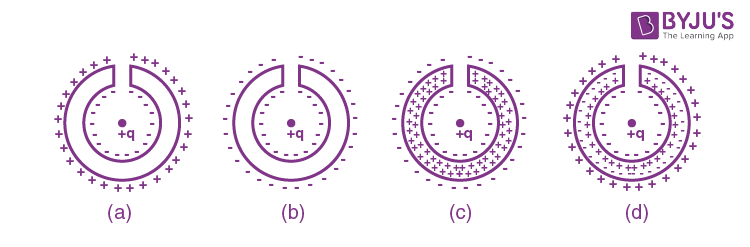Solution:

When a positive charge is placed at the centre of hollow cylindrical conducting shell, an equal amount of negative charge is induced on the inner surface of the shell due to induction process and so an equal amount of positive charge is induced on the outer surface of the shell as shown in option A.

Question 11: A transverse wave of frequency 500 Hz and speed 100 m/s is traveling in the positive x direction on a long string. At time t = 0 s the displacements at x = 0.0 m and at x = 0.25 m are 0.0 m and 0.02 m, respectively. The displacement at x = 0.2 m at t = 5 × 10–4 s is

1. a. –0.04 m
2. b. –0.02 m
3. c. 0.04 m
4. d. 0.02 m

Solution:

Wavelength is λ = v/f = 100/500 = 0.2 m

Let the wave equation be,

y = Asin(kx – ωt + φ) …(1)

Where, ωt + φ = phase

Given,

y = 0 at t = 0, x = 0

0 = Asin (φ)

=> φ = 0

Also, y = 0.02 at t = 0, x = 0.25

Put the values in eq.(1)

0.02 = Asin (2πx/ λ)

0.02 = Asin (2π×0.25/0.2)

=> A = 0.02 m

At t = 5 × 10–4 sec and x = 0.2 m

Again put these values

y = 0.02 sin ((2π×0.2/0.2)-2 π×5×10-4×500)

= 0.02 sin (2 π- π/2)

y = – 0.02 m

Question 12: A thin piece of thermal conductor of constant thermal conductivity insulated on the lateral sides connects two reservoirs which are maintained at temperatures T1 and T2 as shown.

Assuming that the system is in steady-state, which of the following plots best represents the dependence of the rate of change of entropy of the ratio of temperatures T1/T2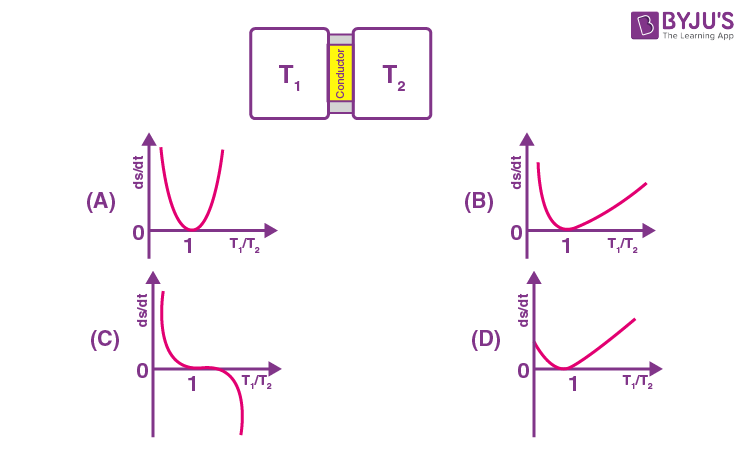Solution:

Let T1> T2 and R is thermal resistance and apply entropy change in the process,

∆S = –(∆Sreservoir – ∆Ssink)

= – (Q/T1)-(Q/T2)

Since Q is the same since the system is at steady state.

∆S = –Q (1/T1 )-(1/T2)

dS/dt = –dQ/dt((1/T1)-(1/T2))

since V = IR

(T1 – T2) = QR

Q = (T1-T2)/R

Put the values of Q in ∆S

Since dS/dt = – ((T1-T2)/R) (T2-T1)/T1T2

dS/dt = ((T2-T1)2/T1T2 )(1/R)

dS/dt = (T12+T22-2T1T2)/T1T2(1/R)

dS/dt = ((T1/T2 )+(T2/T1 )-2)(1/R)

Let T1/T2 = x

dS/dt = (x+(1/x)-2)(1/R)

So, the graph between rate of change of entropy

and ratio of temperatures T1/T2 is,

And it is the graph of (x+(1/x)-2) .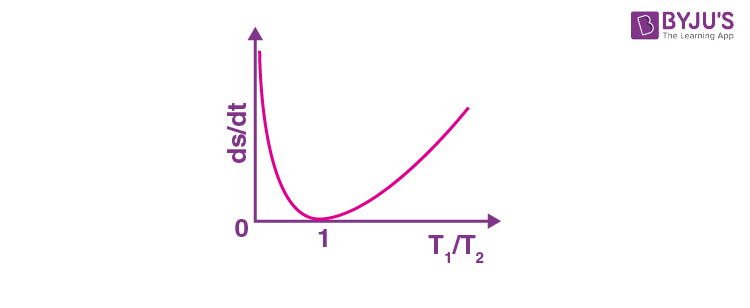Question 13: Which of the following plots represents schematically the dependence of the time period of a pendulum if measured and plotted as a function of its oscillations?

(Note: Amplitude need not be small)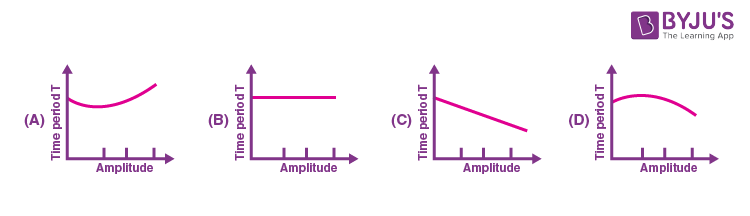Solution:

Time period versus amplitude (θ),

Generally time period is

T = 2π√l/g

l = length of the pendulum, g = acceleration due to gravity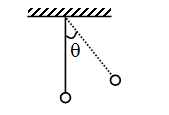(Valid only when θ is very very small)

But the original formula when θ is large

Time period, T = 2π√l/g(1+(1/16) θ02+(11/3072) θ04+…)

So time period of a simple pendulum when θ0 (amplitude) is large is given by

T = 2π√l/g(1+( θ02/16))So, according to this relation time period will increase when the amplitude increase.

T Proportional to 1+( θ02/16).Question 14: On a pulley of mass M hangs a rope with two masses m1 and m2 (m1> m2) tied at the ends as shown in the figure. The pulley rotates without any friction, whereas the friction between the rope and the pulley is large enough to prevent any slipping. Which of the following plots best represents the difference between the tensions in the rope on the two sides of the pulley as a function of the mass of the pulley?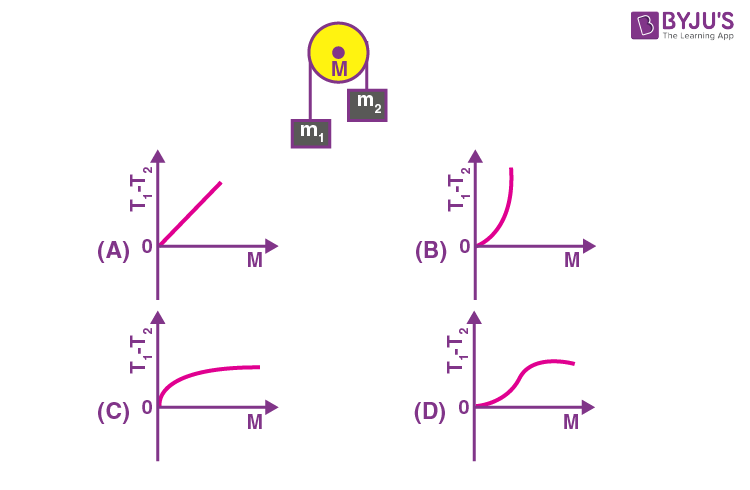Solution: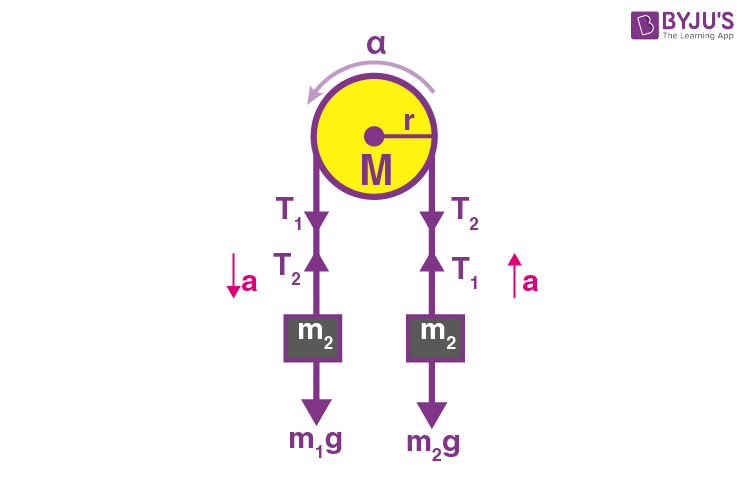Let the tension in both ropes be T1 and T2 respectively and acceleration of masses be a as shown in the figure.

For block m1:

m1a = m1g – T1 …(1)

For block m2:

m2a = T2 – m2g …(2)

Adding eq.(1) & (2), we get

(m1 + m2)a = (m1 – m2)g – (T1 – T2) …(a)

Since Torque, τ = I α

I = moment of inertia, α = angular acceleration

So (T1 – T2)r = (1/2) Mr2 α (Since the moment of inertia of disc I = MR2/2 )

(T1 – T2) = (1/2)Mr α = (1/2) Ma (since a = r α) …(b)

From eq.(a) & (b),

(m1 + m2) ×2(T1-T2)/M = (m1– m2)g – (T1 – T2)

=>T1 – T2 = (m1– m2)g/(1+2(m1 + m2)/M)

Question 15: Two satellites S1 and S2 are revolving around a planet in the opposite sense in coplanar circular concentric orbits. At time t = 0, the satellites are farthest apart. The periods of revolution of S1 and S2 are 3 h and 24 h respectively. The radius of the orbit of S1 is 3 × 104 km. Then the orbital speed of S2 as observed from

1. a. the planet is 4 π × 104 km h–1 when S2 is closest from S1.
2. b. the planet is 2 π × 104 km h–1 when S2 is farthest from S1.
3. c. S1 is π × 104 km h–1 when S2 is closest from S1.
4. d. S1 is 3 π × 104 km h–1 when S2 is closest from S1.

Solution:

From Kepler’s law, T2 proportional to r3

R13/R23 = T12/T22 = 3×3/24×24

=> (3×104/R2)3 = 1/64

3×104/R2 = 1/4

=> R1/R2 = 1/4

R2 = 12 ×104 km

Relative to the planet,

v1 = 2πR1/T1 = 2π×3×104/3 = 2 π×104 km/hr

v2 = 2πR2/T2 = 2π×12×104/24 = π × 104 km/hr

At closest approach distance between S1and S2, the relative orbital speed of S2 as seen from S1 will be v1 + v2 as they are moving in the opposite directions.

Hence, the above orbital speed is,

v = v1 + v2 = 3 π × 104 km/hr.

Question 16: A rectangular region of dimensions w × l (w <<l) has a constant magnetic field into the plane of the paper as shown. On one side the region is bounded by a screen. On the other side, positive ions of mass m and charge q are accelerated from rest and towards the screen by a parallel plate capacitor at constant potential difference v< 0, and come out through a small hole in the upper plate. Which one of the following statements is correct regarding the charge on the ions that hit the screen?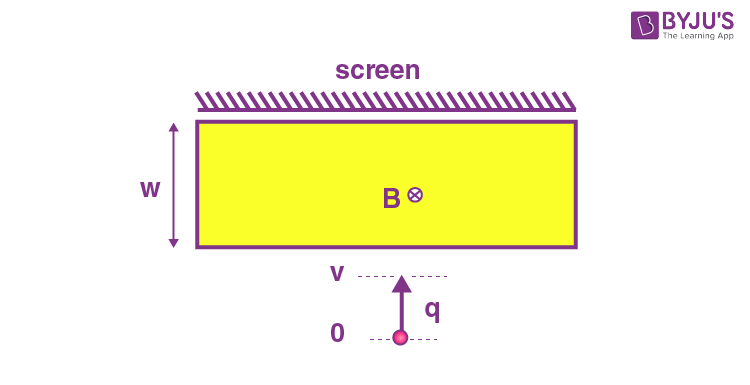1. a. Ions with q >2vm/B2w2 will hit the screen.
2. b. Ions with q <2vm/B2w2 will hit the screen.
3. c. All ions will hit the screen.
4. d. Only ions with q = 2vm/B2w2 will hit the screen.

Solution: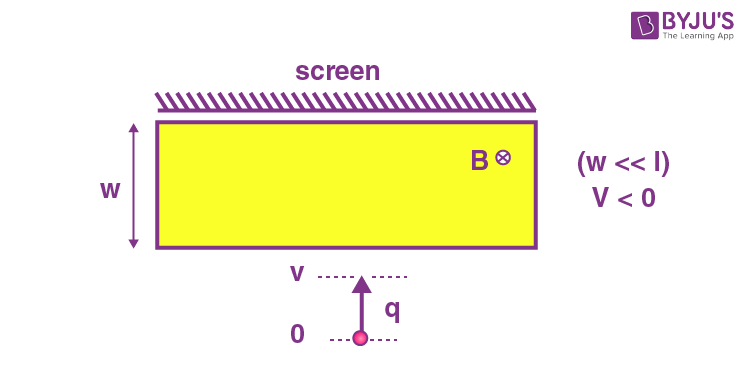Let an ion enters with velocity = v

In the presence of a magnetic field, it will describe circular motion with r = mv/qB

If r < w,

It will make an 1800 turn and come out of the rectangular region having velocity downward.

Question 17: Force $\vec{F}$ applied on a body is written as $\vec{F}=(\hat{n}.\hat{F})\hat{n}+\vec{G}$ , where $\hat{n}$ is a unit vector. The vector $\vec{G}$ is equal to

1. a. $\hat{n}\times \vec{F}$
2. b. $\hat{n}\times (\hat{n}\times \vec{F})$
3. c. $(\hat{n}\times \vec{F})\times \vec{F}/\left | \vec{F} \right |$
4. d. $(\hat{n}\times \vec{F})\times \hat{n}$

Solution:

$(\hat{n}.\vec{F})\hat{n}=0$

Because in scalar tripe product if two vectors are equal, value of scalar tripe product is zero.

$\vec{F}=\vec{G}$

But F = $(\hat{n}\times \vec{F})\times \hat{n}$

Because $\hat{n}$ is unit vector.

$\vec{G}=(\hat{n}\times \vec{F})\times \hat{n}$

Question 18: A particle of mass m moves around the origin in a potential (½)m ω2r2 , where r is the distance from the origin. Applying the Bohr model in this case, the radius of the particle in its nth orbit in terms of a = √(h/(2πmω) is:

1. a. a√n
2. b. an
3. c. an2
4. d. an√n

Solution:

Relation between force and potential energy is given by

Since F = –dU/dr

F = – d(1/2)mv2/dr

Since v = rω

But if r >ω, it hits the screen.

Therefore mv/qB >ω (hits the screen)

=> q < mv/qB …(1)

Since, charge q accelerated by a potential difference of V volt

(1/2)mv2 = qV

v = √(2qV/m)

Put the value of v in eq.(1)

Therefore q < m√(2qV/m)(1/ ωB) .

√q < √(2mV)/ ωB

q < 2mV/ ω2B2 (hit the screen).

Question 19: Two bottles A and B have radii RA and RB and heights hA and hB respectively with RB = 2RA and hB = 2hA. These are filled with hot water at 600C. Consider that heat loss for the bottles takes place only from side surfaces. If the time the water to cool down to 500C is tA and tB for the bottles A and B, respectively, then tA and tB are best related as

1. a. tA = tB
2. b. tB = 2tA
3. c. tB = 4tA
4. d. tA = tA/2

Solution:

From Newton’s law of cooling

dQ/dt = hA ∆T

Assuming, constant rate of cooling,

∆Q = hAT∆t

∆QA/ ∆QB = AAtA/ABtB

Therefore Q = mS∆T

Here, Q = heat, m = mass, S = specific heat and ∆T = change in temperature

mAS∆T/ mBS∆T = AAtA/ABtB (because density = mass/volume)

ρVA/ ρVA = AA tA/ABtB (therefore V = Al)

hAAA/hBAB = AA tA/ABtB

tA/tB = 1/2

tB = 2tA

Question 20: The number of gas molecules striking per second per square meter of the top surface of a table placed in a room at 200C and 1 atmospheric pressure is of the order of (kB = 1.4 ×10–23 J/K, and the average mass of an air molecules is 5 × 10–27 kg)

1. a. 1027
2. b. 1023
3. c. 1025
4. d. 1029

Solution:

Force exerted by the gas molecules on unit area of table, F = PoA = 105 × 1 = 105 N

Average velocity of gas molecules, v = √(8KBT/πm)

Where, T = 20 + 273 = 293 K

So V = √(8×1.4×10-23×293/π×5×10-27 = 1.4×103m/s

Rate of change in momentum ∆P/∆t = nm(v – (–v)) = 2 mnv

Using F = ∆P/∆t = 2 mnv

105 = 2 × 5 × 10–27 × n × 1.4 × 103

=> n = 7 × 1027

So F = -d((1/2)mω2r2 /dr = –(1/2) mω2 d(r)2/dr

=> F = –(1/2) m ω2 (2r)

F = –mω2r

Now, mv2/r = m ω2r …(1)

And by using Bohr’s quantization,

mvr = nh/2 π …(2)

Where, h = Planck’s constant, r = radius, m = mass, n = integer and ω = angular speed

From equation (1) and (2)

(m/r)(nh/2πmr)2 = mω2r

n2h2/(2πm)2ω2 = r4

r = √(nh/2πmω)

since a = √(h/2πnω)

r = a√n.

Question 21: One end of a rod of length L=1m is fixed to a point on the circumference of a wheel of radius $R=\frac{1}{\sqrt{3}}$ . The other end is sliding freely along a straight channel passing through the center O of the wheel as shown in the figure below. The wheel is rotating with a constant angular velocity ω about O.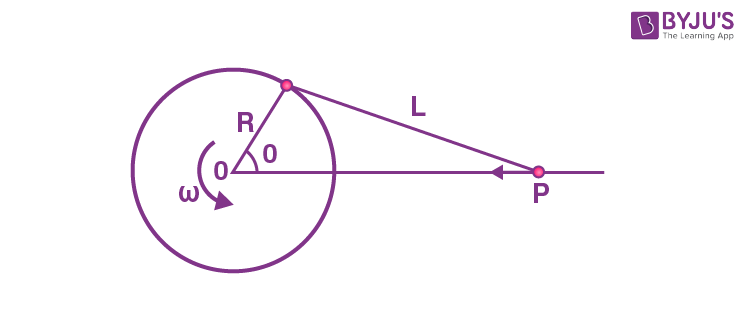The speed of the sliding end P when θ =600 is

1. a. $\frac{2\omega }{3}$
2. b. $\frac{\omega }{3}$
3. c. $\frac{2\omega }{\sqrt{3}}$
4. d. $\frac{\omega }{\sqrt{3}}$

Solution:

Given: L = 1 m

$R=\frac{1}{\sqrt{3}}m$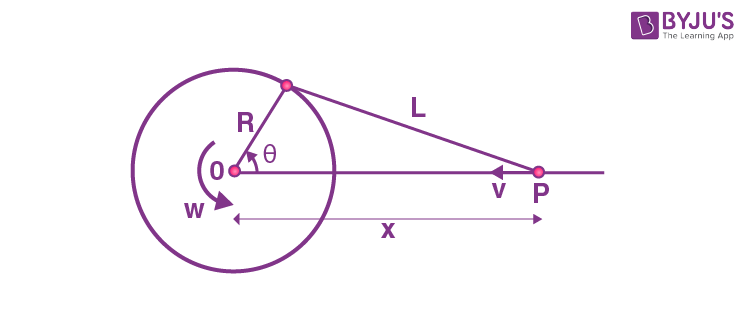It is slider crank mechanism and in it v = –dx/dt

From cosine rule (since L, R $\rightarrow$constant)

$cos\theta =\frac{R^{2}+x^{2}-L^{2}}{2Rx}$

$\Rightarrow R^{2}+x^{2}-L^{2} = 2Rx cos\theta$

Differentiating with respect to time in both the sides.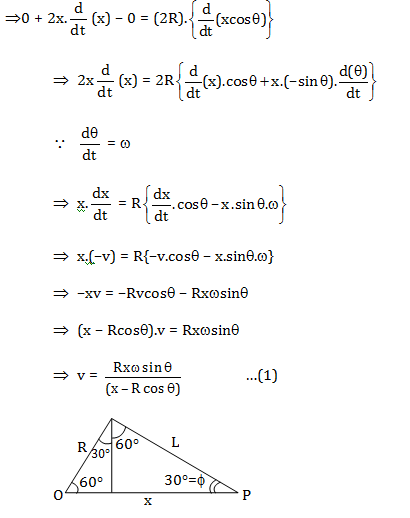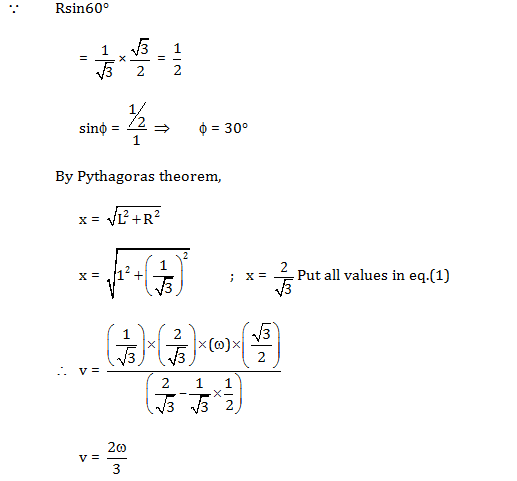Question 22: One mole of an ideal monatomic gas undergoes the following four reversible processes:

Step-1: It is first compressed adiabatically from volume V1 to 1m3.

Step-2: then expanded isothermally to volume 10 m3.

Step-3: then expanded adiabatically to volume V3.

Step-4: then compressed isothermally to volume V1.

If the efficiency of the above cycle is 3/4 then V1 is,

1. a. 2m3
2. b. 4m3
3. c. 6 m3
4. d. 8 m3

Solution:

Here, v1 = v1

v2 = 1 m3

v3 = 10 m3

v4 = v4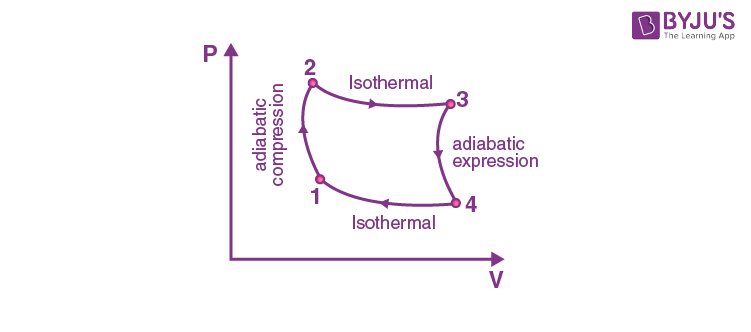Since, efficiency

$\eta =1-\frac{T_{2}}{T_{1}}$

$\frac{T_{2}}{T_{1}}=1-\eta$

$\frac{T_{2}}{T_{1}}=1-\frac{3}{4}=\frac{1}{4}$

For the path, 1 $\rightarrow$ 2 (adiabatic process)

$\frac{T_{1}}{T_{2}}=\left [ \frac{v_{1}}{v_{2}} \right ]^{\gamma -1}$ (for monoatomic γ= 5/3)

4 = (v1)2/3

V1= 8 m3

Question 23: A neutron star with a magnetic moment of magnitude m is spinning with angular velocity ω about its magnetic axis. The electromagnetic power P radiated by it is given by μ0xmy ωzcu where μ0 and c are the permeability and speed of light in free space, respectively. Then

1. a. x = 1, y = 2, z = 4 and u = –3
2. b. x = 1, y = 2, z = 4 and u = 3
3. c. x = –1, y = 2, z = 4 and u = –3
4. d. x = –1, y = 2, z = 4 and u = 3

Solution:

According to question,

p = μ0xmy ωzcu …(1)

Where,

Power, p =work/time = $\frac{M^{1}L^{2}T^{-2}}{T^{1}}=\left [ M^{1}L^{2}T^{-3} \right ]$ = [M1L2T–3]

Angular velocity, ω = rad/sec = [T–1]

Permeability of free space, µ0 = [MLT–2I–2]

m = [IL2]

Speed of light, c = [LT–1]

Put all the dimension is equation (1),

[M1L2T–3] = [MLT–2I–2]x[IL2]y[T–1]z[LT–1]u

[M1L2T–3I0] = Mx.L x+2y+u.T–2x–z–u.I–2x+y

Now compare all the powers,

x = 1 …(2)

x + 2y + u = 2 …(3)

–2x – z – u = –3 …(4)

–2x + y = 0 …(5)

Put value of x in eq.(5)

–2(1) + y = 0

⇒ y = 2

Put the value of x and y in eq.(3)

(1) + 2(2) + u = 2

⇒ u = –3

Put the value of x and u in eq.(4)

–2(1) – z – (–3) = –3

⇒ z = 4

Question 24: A solid cube of wood of side 2a and mass M is resting on a horizontal surface as shown in the figure. The cube is free to rotate about a fixed axis AB. A bullet of mass m (<<M) and speed v is shot horizontally at the face opposite to ABCD at a height 4a/3 from the surface to impart the cube an angular speed ω. It strikes the face and embeds in the cube. Then ωc is close to (Note: The moment of inertia of the cube about an axis perpendicular to the face and passing through the centre of mass is 2Ma2/3)1. a. Mv/ma
2. b. Mv/2ma
3. c. mv/Ma
4. d. mv/2Ma

Solution: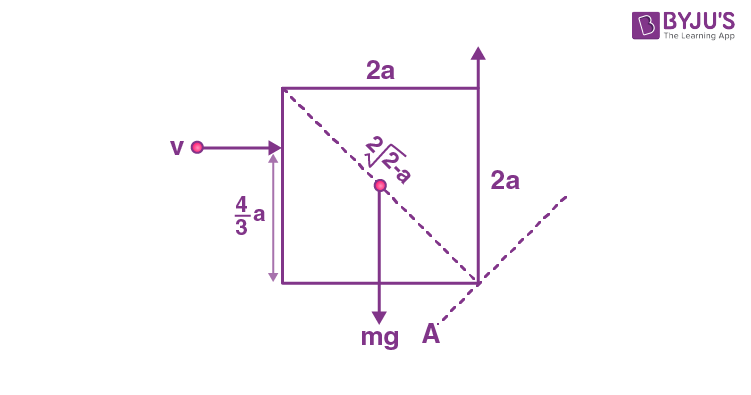Impulsive force is of large magnitude for a very-very small interval of time.

We can neglect mg for a very short interval of time and also the reason for neglecting mg is that impulsive force is of large magnitude as compared to mg.

Angular momentum will be conserved about AB axis,

$mv.\frac{4a}{3}=Iw$ --------------------------(1)

Let coordinates of COM will not change and using parallel axis theorem,

IAB = ICOM + Mr2

Moment of inertia of the cube =2Ma2/3 (Given)

$\Rightarrow I_{AB}=\frac{2Ma^{2}}{3}+M\left [\frac{2\sqrt{2} a}{2} \right ]^{2}$

$\Rightarrow I_{AB}=\frac{2Ma^{2}}{3}+2Ma^{2}$

$\Rightarrow I_{AB}=\frac{8Ma^{2}}{3}$

Put the value in equation (1),

$mv.\frac{4a}{3}=\frac{8Ma^{2}}{3}\omega$

$\Rightarrow mv=2Ma.\omega$

$\Rightarrow \omega =\frac{mv}{2Ma}$

Question 25: A gas obeying the equation of state PV = RT undergoes a hypothetical reversible process described by the equation, $PV^{5/3}exp\left [ -\frac{PV}{E_{0}} \right ]=c_{1}$ where c1 and E0 are dimensioned constants. Then, for this process, the thermal compressibility at high temperature

1. a. Approaches a constant value
2. b. is proportional to T
3. c. is proportional to T1/2
4. d. is proportional to T2

Solution:

Given equation is,

$PV^{5/3}exp\left [ -\frac{PV}{E_{0}} \right ]=c_{1}$

Here, P = pressure, V = volume

Where, C1 and E0 are dimensional constants.

$PV^{\frac{5}{3}}.\frac{1}{e^{(\frac{PV}{E_{0}})}}=C_{1}$

By taking loge both the sides,

ln(PV 5/3) = ln[C1.ePV/Eo]

Since, ln(AB) = ln A + ln B

and lnAB = B lnA

Therefore, ln P + lnV5/3 = lnC1 + lnePV/Eo

ln P +(5/3) lnV = lnC1 + (PV/E0) lne

ln P + (5/3) lnV = lnC1 + PV/E0

Since, logee = 1

Differentiating both the sides with respect to t,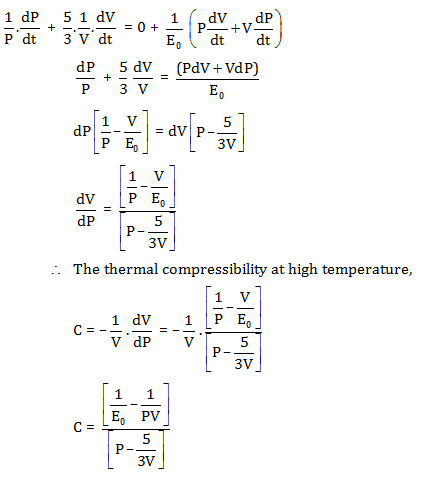Question 26: To calculate the size of a hydrogen anion using the Bohr model, we assume that its two electrons move in an orbit such that they are always on diametrically opposite sides of the nucleus. With each electron having the angular momentum h = h/2 π, and taking electron interaction into account the radius of the orbit in terms of the Bohr radius of hydrogen atom $a_{E}=\frac{4\pi \varepsilon _{0}h^{2}}{me^{2}}$ is:

1. a. aB
2. b. (4/3)aB
3. c. (2/3) aB
4. d. (3/2) aB

Solution: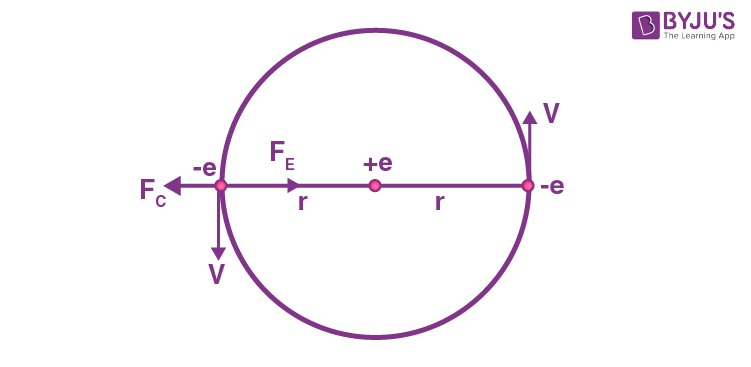Let the velocity of the electron be v.

Angular momentum of electron,

mvr = h (given)

Net electrostatic force acting on the electron,

$F_{e}=\frac{e^{2}}{4\pi \varepsilon _{0}r^{2}}-\frac{e^{2}}{4\pi \varepsilon _{0}2r^{2}}$

= $F_{e}=\frac{3}{4}\frac{e^{2}}{4\pi \varepsilon _{0}r^{2}}$

$Centrifugal force,F_{c}=\frac{mv^{2}}{r}=\frac{(mvr)^{2}}{r\times mr^{2}}=\frac{h^{2}}{mr^{3}}$

But, Fc = Fe

Therefore $\frac{h^{2}}{mr^{3}}=\frac{3}{4}.\frac{e^{2}}{4\pi \varepsilon _{0}r^{2}}$

$\Rightarrow r =\frac{4}{3}.\frac{4\pi _{0}h^{2}}{me^{2}}=\frac{4}{3}a_{B}$

Question 27: A square-shaped conducting wire loop of dimension a moving parallel to the x-axis approaches a square region of size b (a < b) where a uniform magnetic field B exists pointing into the plane of the paper (see figure). As the loop passes through this region, the plot correctly depicting its speed (v) as a function of x is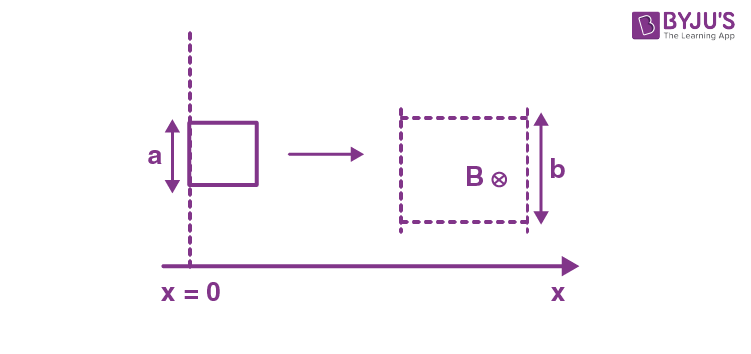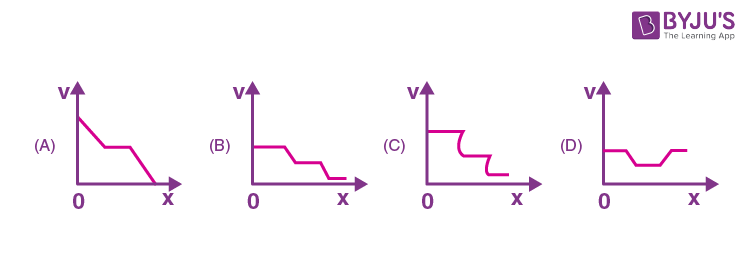Solution: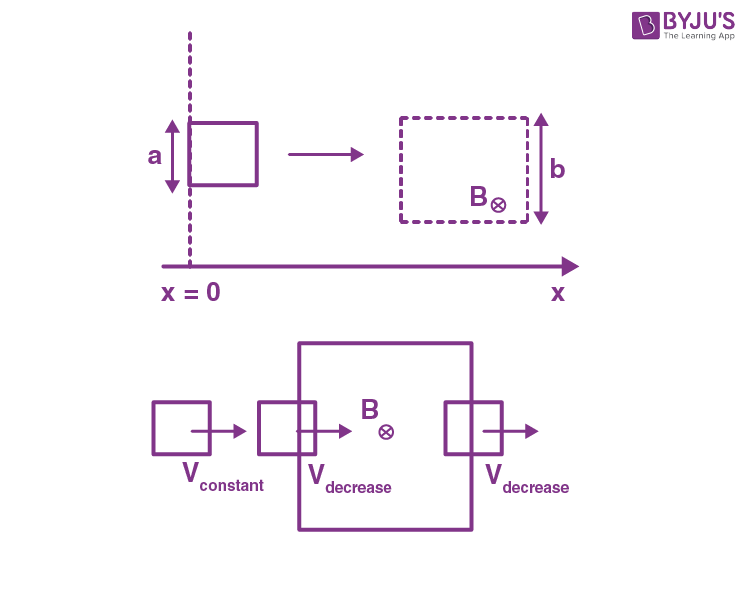Initially, the velocity remains the same. Then when it is about to enter the loop, by Faraday’s law the velocity will decrease and then will remain same until it comes out of the loop, when it exits the loop again by Faraday’s law the velocity will decrease.

Question 28: The figure of a centimetre scale below shows a particular position of the vernier callipers. In this position, the value of x shown in the figure is (the figure is not to scale)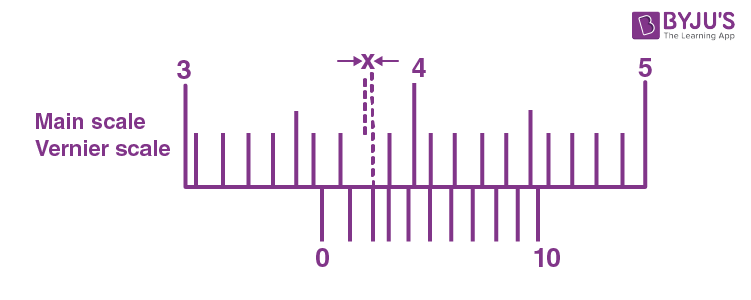1. a. 0.02 cm
2. b. 3.65 cm
3. c. 4.15 cm
4. d. 0.03 cm

Solution:

At the 5th reading of verniers scale, both readings of the scales coincide.

At the 4th reading of vernier’s scale, the length between the two scales is minimum and will be the least count = 0.01 cm

So difference at 3rd reading of the vernier scale is 2 × 0.01 cm

The difference at 2nd reading of vernier scale will be

= 3 × 0.01 cm = 0.03 cm

Question 29: A parallel beam of light is incident on a tank filled with water up to a height of 61.5 mm as shown in the figure below. Ultrasonic waves of frequency 0.5 MHz are sent along the length of the water column using a transducer placed at the top, and they form longitudinal standing waves in the water. Which of the schematic plots below best describes the intensity distribution of the light as seen on the screen?

Take the speed of sound in water to be 1,500 m/s.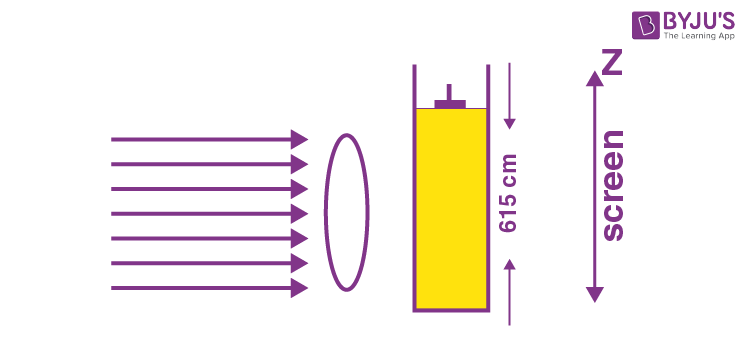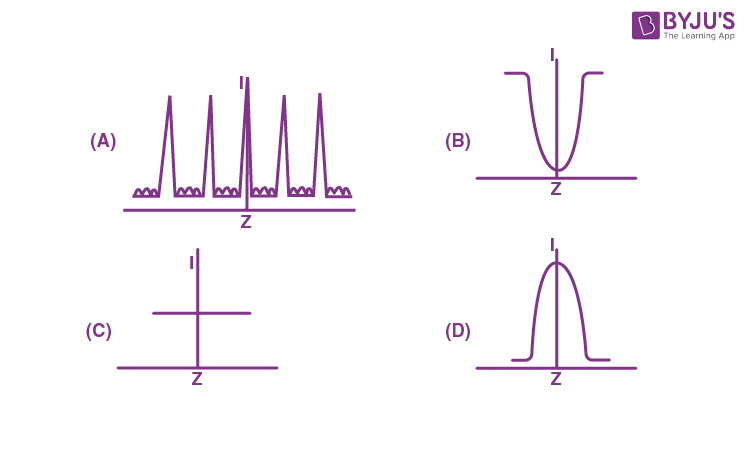Solution:

As the rays pass through water, there will be a phase difference between the two consecutive emerging rays thereby forming a pattern similar to YDSE.

Since, We know that,

Velocity = frequency × wavelength

v = f λ

$\Rightarrow \lambda =\frac{1500}{0.5\times 10^{6}}=3000\times 10^{-6}$

λ=3 x 10-3m

⇒ λ=3 mmIntensity vary according to graph ‘A’.

Question 30: A star of mass M (equal to the solar mass) with a planet (much smaller than the star) revolves around the star in a circular orbit. The velocity of the star with respect to the centre of mass of the star-planet system is shown below: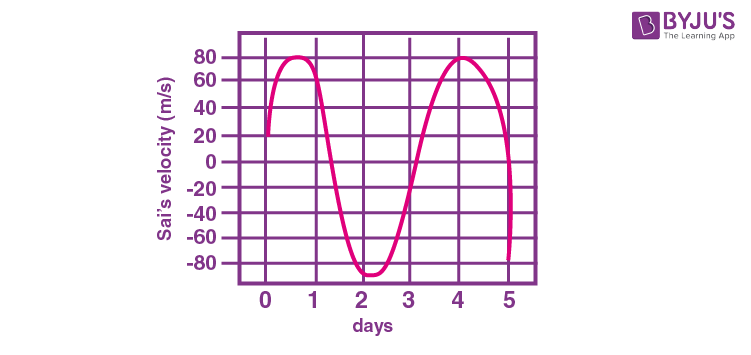The radius of the planet's orbit is closest to (1 A. U. = Earth-Sun distance)

1. a. 0.004 A. U.
2. b. 0.008 A.U.
3. c. 0.004 A.U.
4. d. 0.12 A.U.

Solution:

From Kepler’s-3rd law, we have the square of time period that is directly proportional to the cube of radius of the orbit.

Let T1 = time period of planet

from graph,

T1 = 3 days

T2 = Time period of earth = 365 days

r1 = radius of planet orbit

r2 = radius of earth orbit

r2 = 1 A.U. (given)

By Kepler’s law, we have

$\frac{(T_{1})^{2}}{(T_{2})^{2}}=\frac{(r_{1})^{3}}{(1A.U.)^{3}}$

$\Rightarrow\left [ \frac{3}{365} \right ]^{2}=\frac{(r_{1})^{3}}{(1A.U)^{3}}$

$\Rightarrow\left [ \frac{3}{365} \right ]^{2/3}=\frac{(r_{1})}{(1A.U)}$

$\Rightarrow 0.04 =\frac{r_{1}}{1A.U.}$

$\Rightarrow r_{1}=0.04 A.U.$

### KVPY SX 2017 Physics Paper with Solutions